# A Beginner’s Guide: Private and Public Key Cryptography Deciphered

This article will explain at a high-level Private and Public Key Cryptography used in Bitcoin and it’s unique security feature.

We will be looking at how Public Keys are generated, why this is secure and how Private Keys are linked to Public Keys.

### Why?

• Provide a high-level, easy to digest explanation of a complex topic
• Greater insight and understanding on how Bitcoin derives some of its security features
• By generating a Public Key, we have a way to interact with other people on the network openly, for example we may have some Bitcoin “attached” to our Public Key
• The only way for us to prove that we own that Bitcoin is to prove that the Public Key was derived from our Private Key (by providing a signature, beyond the scope of this article)We are going to make this simple to understand

### What is a Private Key?

• A private key, is simply an extremely large and highly random number
• The image below shows a Private Key generated by a wallet program written by me
• DO NOT USE THIS NUMBER AS YOUR PRIVATE KEY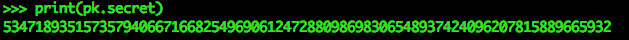Generated Private Key

### What is a Public Key?

• Public Keys can be shared with other people on the network
• We can generate addresses from the Public Key
• Public Keys are used by other users on the network to challenge ownership of Bitcoins
• This is a generated Public Key from our Private Key above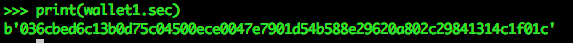Public Key shown as hexadecimal representation of bytes

### How are Public Keys generated?

• From a Private Key we can generate a Public Key
• The Public Key is an (x ,y) co-ordinate on an Elliptic Curve
• The Public Key would be any point on this curve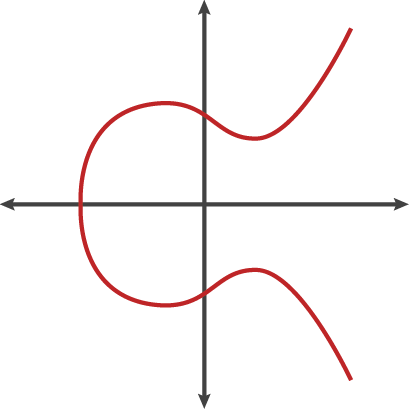Elliptic Curve

• The Private Key is used as a scalar (All this means is that Private Key Number is used as a multiplier)
• All we need to know for now, is that there is a publicly known point on this curve, lets call it G
• G will be our starting position and has an (x, y) co-ordinate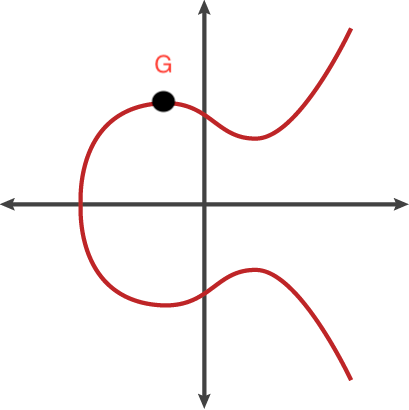G is a publicly known point

• What we do next is multiply the (x, y) co-ordinate G, by itself Private Key (number) of times
• In other words, we are performing G*G, Private Key number of times
• When this occurs, there is a pin balleffect, this point G will bounce around the Elliptic Curve, like in a pin ball machine, hitting another point on the curve, reflecting over the x-axis and this is repeated * Private Key (number) of times
• Once this process is complete, we have our Public Key, viola!
• Our Public Key is nothing more than a (x, y) co-ordinate on a curve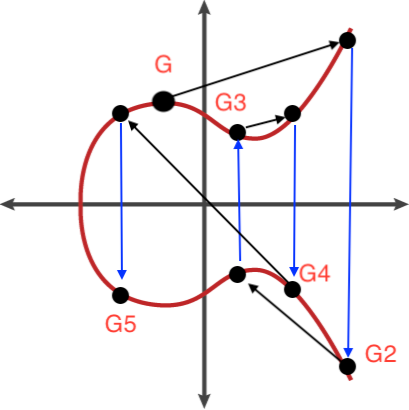“Pin Ball” Effect, Point G is bounced around the curve

### How does this provide security?

• Ok so we’ve simplified some complex topics
• For a more detailed look at Elliptic Curves and the Mathematics behind them here is a link to my next article <Watch this space for a new article>
• The only way our Public Key, therefore our Bitcoin can be comprised is by revealing our Private Key

#### Let’s do an Experiment

• In the image below, we can see a billiards table and the starting position of the cue ball
• Lets say this starting position is our point G on the Elliptic CurveOur starting position G

• After knocking the cue ball around the billiards table we stop at our final position
• Let’s say this final position is our (x, y) position on the Elliptic Curve, making it our Public KeyOur final position, the Public Key point
• Can you tell me from looking at the starting position of the cue ball (G) and the final position of the cue ball (Public Key)…
• How many times did the cue ball hit the cushions?
• Please try and guess before looking at the answer…

• I have video evidence… (Yes I did spend time knocking that cue ball around)
• The answer is 36

### The Discrete Log Problem

• Using the experiment above, we can demonstrate a key security feature of Private and Public Key Cryptography known as The Discrete Log Problem
• By knowing the starting position (G) and the final position (Public Key) we cannot deduce the scalar (Private Key), given we are dealing with extremely large numbers
• This equation cannot be reversed, also known as a Trap-Door Function or a One Way Function, given publicly known information
• It’s easy to create the Public Key given the Private Key
• But it’s extremely difficult to calculate the Private Key from the Public Key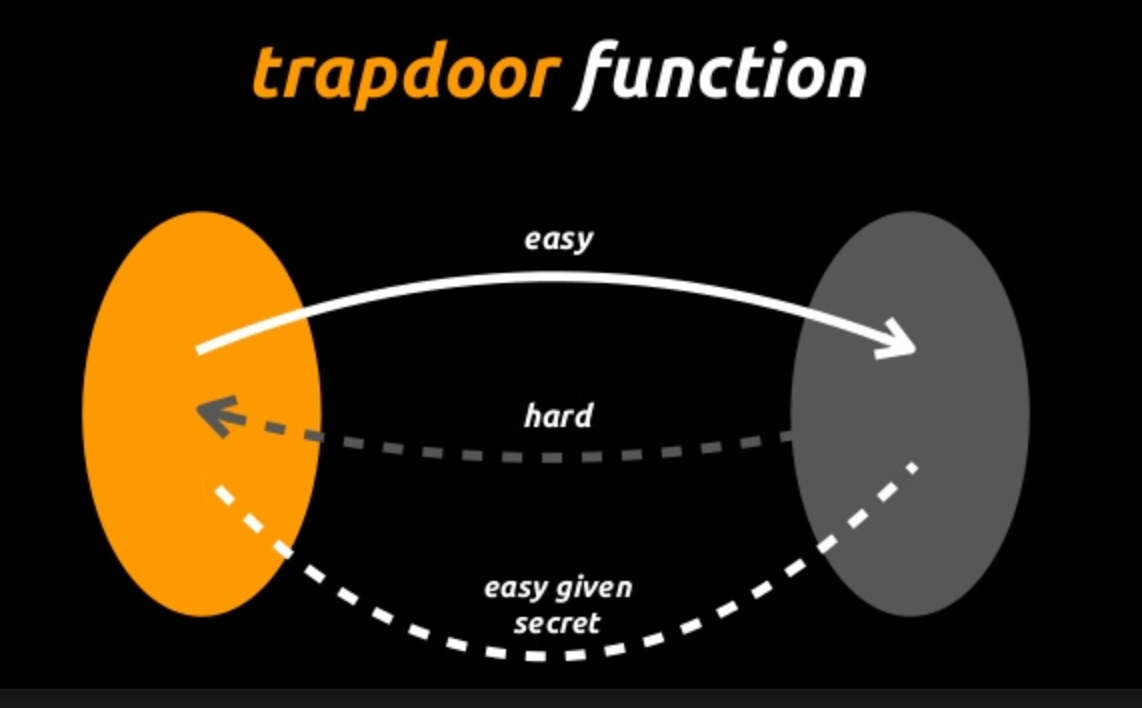Trap-door function

### Conclusion

• Private keys are simply extremely large and random numbers
• Public Keys are (x, y) points on an Elliptic Curve, generated by using the Private Key as a scalar
• Private and Public Key cryptography derives its security from the Discrete Log Problem, given the starting value and the end value, it’s difficult to deduce the scalar (Private Key)
• Trap-Door Functions are functions that cannot be reversed, it’s easy to create the Public Key given the Private Key but extremely difficult to calculate the Private Key from the Public Key

Knowledge gained in this article was from Jimmy Song’s Programming Blockchain 2-day Seminar.

I highly recommend this course to any programmer serious about cutting their teeth into Bitcoin and Blockchain Technology (Not for the faint of heart)

#### Interested in going further down the rabbit hole?

<Learn about Elliptic Curves and the Maths behind them>